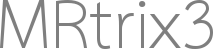# logFC regression with fixelcfestats

Hey MRtrixperts,

I’ve ran a simple age regression analysis in the FBA pipeline with a contrast that looks like:

0 1

and a design matrix that looks something like

1 26.34
1 3.34
1 3.34
1 -10.65
1 -12.65

To present the information it would be useful to have a change in FDC/FD/FC per year/decade.
I assume for FD & FDC if you take beta1.mif and multiply by 100 this would give change per year per fixel.

mrcalc beta1.mif 100 -mult chg_per_year.mif

my question is though, since logFC is used instead of FC, how would you calculate the change per year of FC?
If my assumptions are correct then beta1 would be equal to log(FC)/age, and taking the exponential of beta1 would yield FC*e^(1/age).

Is there a way to do this? Are my assumptions correct?

Thanks for all the help

Matt

Hi Matt,

MRtrixperts

Terminology adopted.I assume for FD & FDC if you take beta1.mif and multiply by 100 this would give change per year per fixel.

This depends on the values that have been placed into the design matrix. If these values are in years (de-meaned), then the corresponding beta value represents the regressed change in your quantitative value per year. Hence there would be no need for any multiplicative scaling.

since logFC is used instead of FC, how would you calculate the change per year of FC?

You’re correct that beta1 will encode the change in `log(FC)` per year. If the quantity you want to report is the change in `FC` per year, then the conversion you require is that between `log(FC)` and `FC`. Assuming there’s no global drift in population template generation, the mean value of `FC` across all participants should be 1.0, and the mean value of `log(FC)` across participants should be 0.0. Moreover, since the derivative of `log(x)` at `x`=1 is unity, any infintessimal change in `log(FC)` at `log(FC)=0.0` will be directly echoed in `FC`. So my instinct is to report the value of beta1 as the change in `FC` per year, without any algebraic manipulation to account for the fact that the calculations were in fact performed on `log(FC)`.

Anyone is free to correct me on this though… There are more complex experimental designs where this approach is not entirely appropriate, but for this relatively simple case this is what makes sense to me.

Rob

Hi Rob,

Thank you for your reply! This is certainly enough justification for me, and makes sense.

Cheers

Matt